# QuTiP Example: Superoperators, Pauli Basis and Channel Contraction¶

Institute for Quantum Computing $\newcommand{\ket}{\left|#1\right\rangle}$ $\newcommand{\bra}{\left\langle#1\right|}$ $\newcommand{\cnot}{{\rm CNOT}}$ $\newcommand{\Tr}{\operatorname{Tr}}$

## Introduction¶

In this notebook, we will demonstrate the tensor_contract function, which contracts one or more pairs of indices of a Qobj. This functionality can be used to find rectangular superoperators that implement the partial trace channel $S(\rho) = \Tr_2(\rho)$, for instance. Using this functionality, we can quickly turn a system-environment representation of an open quantum process into a superoperator representation.

## Preamble¶

### Features¶

We enable a few features such that this notebook runs in both Python 2 and 3.

In :
from __future__ import division, print_function


### Imports¶

In :
import numpy as np
import qutip as qt

from qutip.ipynbtools import version_table


### Plotting Support¶

In :
%matplotlib inline


### Settings¶

In :
qt.settings.colorblind_safe = True


## Superoperator Representations and Plotting¶

We start off by first demonstrating plotting of superoperators, as this will be useful to us in visualizing the results of a contracted channel.

In particular, we will use Hinton diagrams as implemented by qutip.visualization.hinton, which show the real parts of matrix elements as squares whose size and color both correspond to the magnitude of each element. To illustrate, we first plot a few density operators.

In :
qt.visualization.hinton(qt.identity([2, 3]).unit());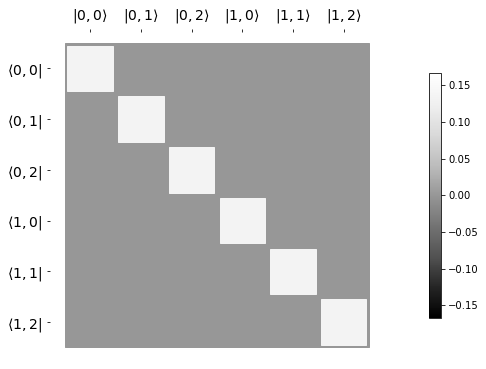In :
qt.visualization.hinton(qt.Qobj([
[1, 0.5],
[0.5, 1]
]).unit());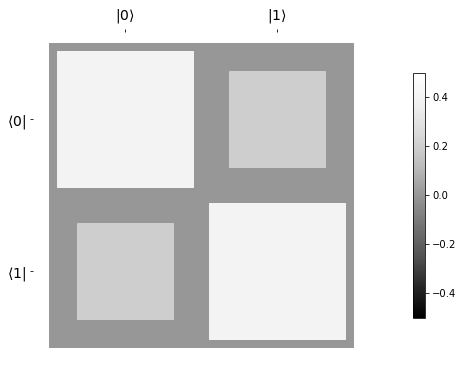We show superoperators as matrices in the Pauli basis, such that any Hermicity-preserving map is represented by a real-valued matrix. This is especially convienent for use with Hinton diagrams, as the plot thus carries complete information about the channel.

As an example, conjugation by $\sigma_z$ leaves $\mathbb{1}$ and $\sigma_z$ invariant, but flips the sign of $\sigma_x$ and $\sigma_y$. This is indicated in Hinton diagrams by a negative-valued square for the sign change and a positive-valued square for a +1 sign.

In :
qt.visualization.hinton(qt.to_super(qt.sigmaz()));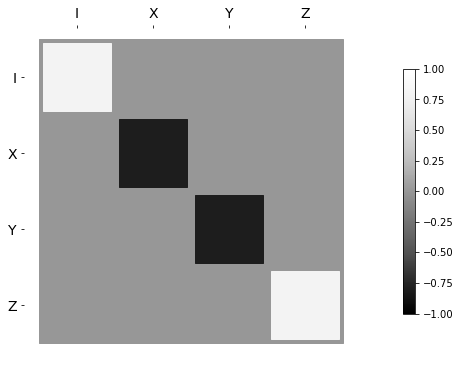As a couple more examples, we also consider the supermatrix for a Hadamard transform and for $\sigma_z \otimes H$.

In :
qt.visualization.hinton(qt.to_super(qt.hadamard_transform()));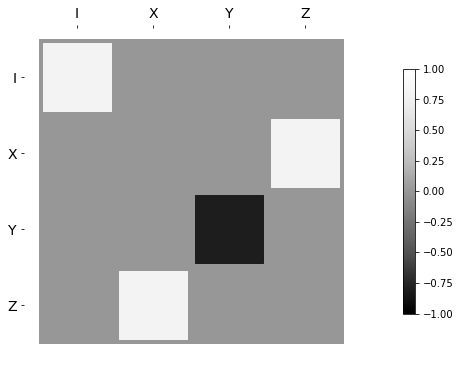In :
qt.visualization.hinton(qt.to_super(qt.tensor(qt.sigmaz(), qt.hadamard_transform())));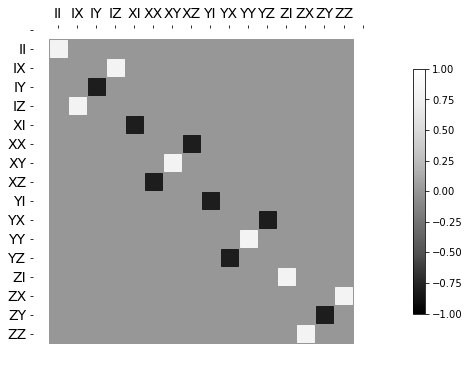## Reduced Channels¶

As an example of tensor contraction, we now consider the map $S(\rho) = \Tr_2[\cnot (\rho \otimes \ket{0}\bra{0}) \cnot^\dagger]$. We can think of the $\cnot$ here as a system-environment representation of an open quantum process, in which an environment register is prepared in a state $\rho_{\text{anc}}$, then a unitary acts jointly on the system of interest and environment. Finally, the environment is traced out, leaving a channel on the system alone. In terms of Wood diagrams, this can be represented as the composition of a preparation map, evolution under the system-environment unitary, and then a measurement map.The two tensor wires on the left indicate where we must take a tensor contraction to obtain the measurement map. Numbering the tensor wires from 0 to 3, this corresponds to a tensor_contract argument of (1, 3).

In :
s_meas = qt.tensor_contract(qt.to_super(qt.identity([2, 2])), (1, 3))
s_meas

Out:
Quantum object: dims = [[, ], [[2, 2], [2, 2]]], shape = (4, 16), type = other\begin{equation*}\left(\begin{array}{*{11}c}1.0 & 0.0 & 0.0 & 0.0 & 0.0 & \cdots & 0.0 & 0.0 & 0.0 & 0.0 & 0.0\\0.0 & 0.0 & 1.0 & 0.0 & 0.0 & \cdots & 0.0 & 0.0 & 0.0 & 0.0 & 0.0\\0.0 & 0.0 & 0.0 & 0.0 & 0.0 & \cdots & 0.0 & 0.0 & 1.0 & 0.0 & 0.0\\0.0 & 0.0 & 0.0 & 0.0 & 0.0 & \cdots & 0.0 & 0.0 & 0.0 & 0.0 & 1.0\\\end{array}\right)\end{equation*}

Meanwhile, the super_tensor function implements the swap on the right, such that we can quickly find the preparation map.

In :
q = qt.tensor(qt.identity(2), qt.basis(2))
s_prep = qt.sprepost(q, q.dag())
s_prep

Out:
Quantum object: dims = [[[2, 2], [2, 2]], [, ]], shape = (16, 4), type = other\begin{equation*}\left(\begin{array}{*{11}c}1.0 & 0.0 & 0.0 & 0.0\\0.0 & 0.0 & 0.0 & 0.0\\0.0 & 1.0 & 0.0 & 0.0\\0.0 & 0.0 & 0.0 & 0.0\\0.0 & 0.0 & 0.0 & 0.0\\\vdots & \vdots & \vdots & \vdots\\0.0 & 0.0 & 0.0 & 0.0\\0.0 & 0.0 & 0.0 & 0.0\\0.0 & 0.0 & 0.0 & 0.0\\0.0 & 0.0 & 0.0 & 0.0\\0.0 & 0.0 & 0.0 & 0.0\\\end{array}\right)\end{equation*}

For a $\cnot$ system-environment model, the composition of these maps should give us a completely dephasing channel. The channel on both qubits is just the superunitary $\cnot$ channel:

In :
qt.visualization.hinton(qt.to_super(qt.cnot()))

Out:
(<matplotlib.figure.Figure at 0x11882c7f0>,
<matplotlib.axes._subplots.AxesSubplot at 0x118b74898>)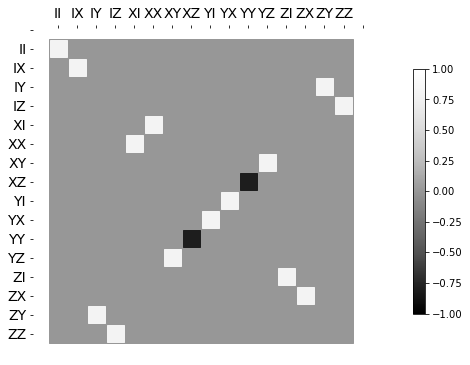We now complete by multiplying the superunitary $\cnot$ by the preparation channel above, then applying the partial trace channel by contracting the second and fourth index indices. As expected, this gives us a dephasing map.

In :
qt.tensor_contract(qt.to_super(qt.cnot()), (1, 3)) * s_prep

Out:
Quantum object: dims = [[, ], [, ]], shape = (4, 4), type = super, isherm = True\begin{equation*}\left(\begin{array}{*{11}c}1.0 & 0.0 & 0.0 & 0.0\\0.0 & 0.0 & 0.0 & 0.0\\0.0 & 0.0 & 0.0 & 0.0\\0.0 & 0.0 & 0.0 & 1.0\\\end{array}\right)\end{equation*}
In :
qt.visualization.hinton(qt.tensor_contract(qt.to_super(qt.cnot()), (1, 3)) * s_prep);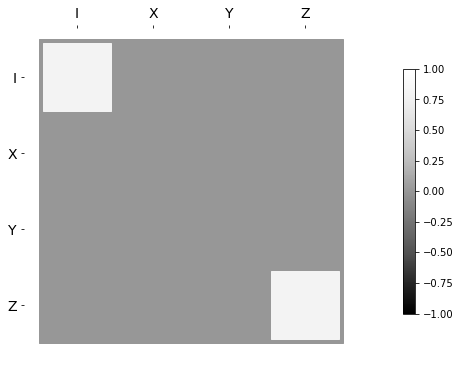## Epilouge¶

In :
version_table()

Out:
SoftwareVersion
QuTiP4.3.0.dev0+6e5b1d43
Numpy1.13.1
SciPy0.19.1
matplotlib2.0.2
Cython0.25.2
Number of CPUs2
BLAS InfoINTEL MKL
IPython6.1.0
Python3.6.2 |Anaconda custom (x86_64)| (default, Jul 20 2017, 13:14:59) [GCC 4.2.1 Compatible Apple LLVM 6.0 (clang-600.0.57)]
OSposix [darwin]
Thu Jul 20 22:59:16 2017 MDT